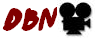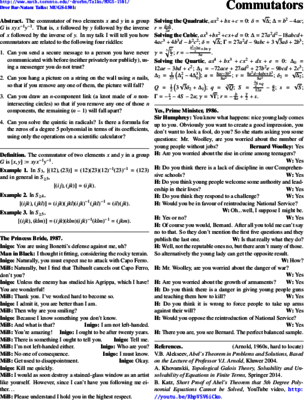Handout: Commutators-Handout.pdf.
Slides: Commutators-Slides.nb.
Talk video:.Sources: pensieve.
© | << < ? > >> | Dror Bar-Natan: Talks:

# Commutators

## Math Union Guest Speaker

### 4PM Friday January 16 2015, Bahen B024

Abstract. The commutator of two elements $x$ and $y$ in a group $G$ is $xyx^{-1}y^{-1}$. That is, $x$ followed by $y$ followed by the inverse of $x$ followed by the inverse of $y$. In my talk I will tell you how commutators are related to the following four riddles:

1. Can you send a secure message to a person you have never communicated with before (neither privately nor publicly), using a messenger you do not trust?
2. Can you hang a picture on a string on the wall using $n$ nails, so that if you remove any one of them, the picture will fall?
3. Can you draw an $n$-component link (a knot made of $n$ non-intersecting circles) so that if you remove any one of those $n$ components, the remaining $n-1$ will fall apart?
4. Can you solve the quintic in radicals? Is there a formula for the zeros of a degree $5$ polynomial in terms of its coefficients, using only the operations on a scientific calculator?

Prerequisites.

1. The first week of any class on group theory.
2. Knowing that every complex number other than to $0$ has exactly $n$ roots of order $n$, and how to compute them.